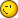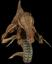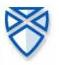7 replies to this topic

### #1mortezahaydari

mortezahaydari

Casio Fan

•• Members
•• 34 posts

• Calculators:
casio fx-991

Posted 19 February 2006 - 12:01 PM

i think that cp300 solve is very bad because
solve((x+1)^2=0) --> {x=-1}
but i want to know how many times it happens...!!
i think OS should upgrade this

### #2Kilburn

Kilburn

Casio Technician

•• Members
•• 491 posts
• Gender:Male
• Location:France
• Interests:Blah

• Calculators:
FX-7500 G

Posted 19 February 2006 - 12:32 PM

i think that cp300 solve is very bad because
solve((x+1)^2=0) --> {x=-1}
but i want to know how many times it happens...!!
i think OS should upgrade this

Well the answer is good, no? (-1+1)^2=0^2=0
What do you mean with "how many times it happens"??? You want to konw how many solutions there are? You should just write dim(solve((x+1)^2=0)), as the returned value is a list!### #3TacoFred

TacoFred

Casio Freak

•• Members
•• 145 posts
• Location:NJ
• Interests:I LOVE STARCRAFT BROODWAR<br />MUHAHAHAHAHAHAHAHAHAHAHA

• Calculators:
cfx 9850gb+, fx 115MS, ClassPad 300, TI-89 Titanium

Posted 20 February 2006 - 03:19 PM

if you mean multiplicity, then since the function is (x+1)^2, the solution is x=-1 with multiplicity 2....so simple

### #4Guest_pabloec20_*

Guest_pabloec20_*
•• Guests

Posted 09 March 2006 - 04:08 PM

i did the dim trick and i get "1" what does it mean? the multiplicity is 2 so its not the multiplicity what i am gettin...
usin os 1 dunno if that has anything to do

### #5SoftCalc

SoftCalc

Casio Technician

•• Members
•• 406 posts
• Location:Portland, OR USA

• Calculators:
ClassPad 300 , AFX 2.0, HP-48/49/50, TI-89/92/Voyager, HP Expander, etc...

Posted 10 March 2006 - 05:55 PM

i did the dim trick and i get "1" what does it mean? the multiplicity is 2 so its not the multiplicity what i am gettin...
usin os 1 dunno if that has anything to do

Because the original equation is quadratic it has two solutions, but they are both the same. The solution is x=-1, but this is a repeated root. I think most algebra systems will not give you the multiplicity of a root.

### #6R00KIE

R00KIE

Casio Freak

•• Members
•• 155 posts
• Location:Portugal
• Interests:Electronics, games, programming

• Calculators:
HP49G ROM 1.24; CASIO CFX-9850GB PLUS;CASIO FX-6300G; CASIO FX-82TL

Posted 10 March 2006 - 07:16 PM

You are right, not many CAS give the multiplicity of a root unless you have a command that returns the roots and it's multiplicity, i have tested it in my hp49 and i needed to use a command that i don't use that often to solve equations to get the root multiplicity, so don't complain that the cas gives wrong results because it doesn't in most cases ans when it does it's because most probably there are better ways to solve the problem

### #7Overlord

Overlord

Casio Technician

•• Moderator
•• 355 posts
• Gender:Male
• Location:Brussels - Belgium
• Interests:Math Researcher

• Calculators:
My head - C300 OS 3.00 - G100 Rom 1.02 - G65 - G60 - G25

Posted 10 March 2006 - 10:43 PM

you can differentiate (x+1)^2, it gives 2(x+1). As -1 is root of (x+1)^2 and of 2(x+1), you can say the multiplicity is at least 2

If a is a root of P(x) with multiplicity m, then a is also a root of dP(x)/dx with multiplicity m-1, d?P(x)/dx? with multiplicity m-2, ...

### #8mortezahaydari

mortezahaydari

Casio Fan

•• Members
•• 34 posts

• Calculators:
casio fx-991

Posted 11 March 2006 - 10:31 AM

you can differentiate (x+1)^2, it gives 2(x+1). As -1 is root of (x+1)^2 and of 2(x+1), you can say the multiplicity is at least 2

If a is a root of P(x) with multiplicity m, then a is also a root of dP(x)/dx with multiplicity m-1, d?P(x)/dx? with multiplicity m-2, ...

i know this idea but there is equations that you can not do this,something like (x+a)^2*(x+b )(x+c)
there could be a x=-a answer 3 times.. at last it is to slow to do this

#### 0 user(s) are reading this topic

0 members, 0 guests, 0 anonymous users